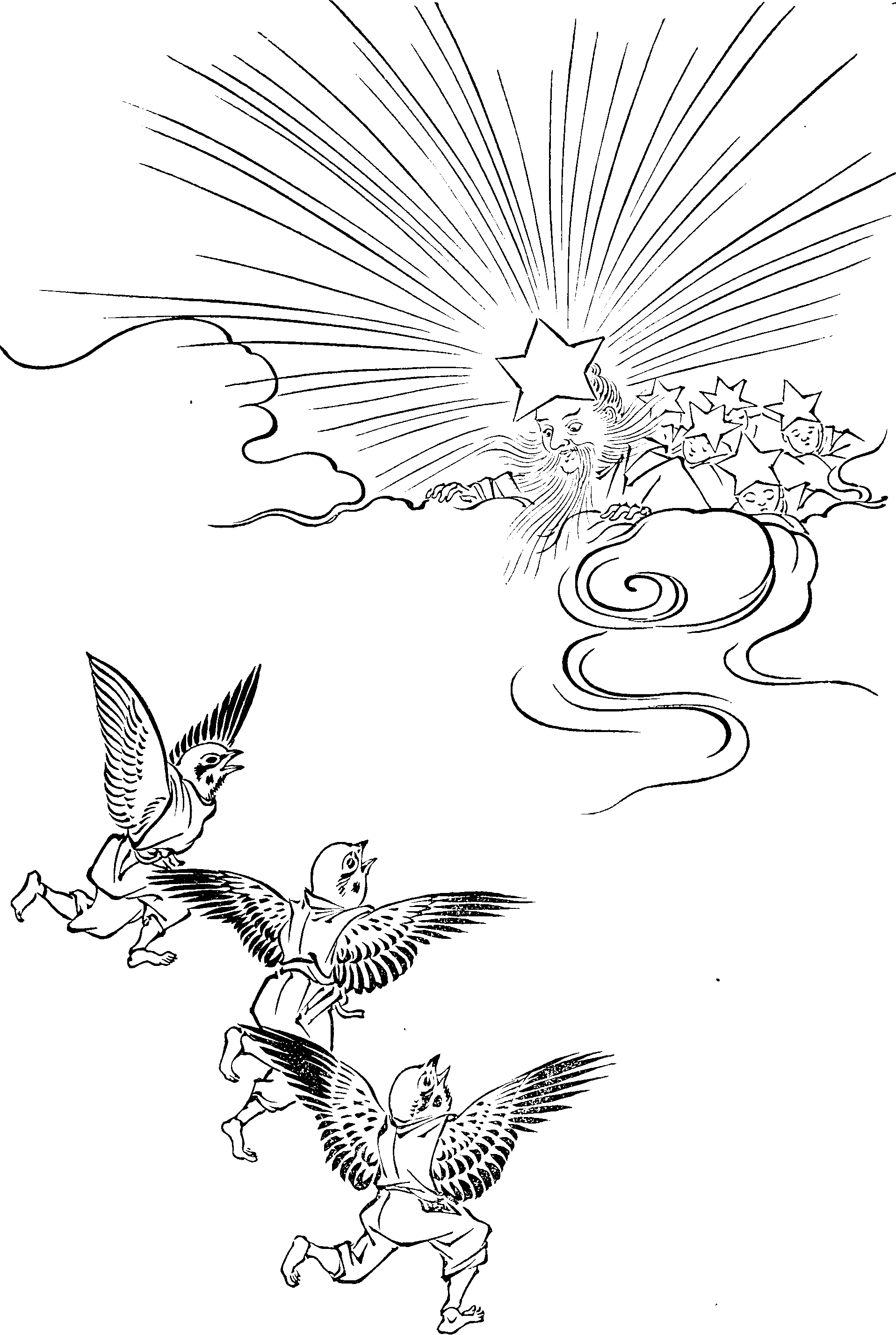# Generalised autoregressive processes

$\renewcommand{\var}{\operatorname{Var}} \renewcommand{\corr}{\operatorname{Corr}} \renewcommand{\dd}{\mathrm{d}} \renewcommand{\bb}{\mathbb{#1}} \renewcommand{\vv}{\boldsymbol{#1}} \renewcommand{\rv}{\mathsf{#1}} \renewcommand{\vrv}{\vv{\rv{#1}}} \renewcommand{\disteq}{\stackrel{d}{=}} \renewcommand{\gvn}{\mid} \renewcommand{\Ex}{\mathbb{E}} \renewcommand{\Pr}{\mathbb{P}}$

Some useful generalisations of autoregressive (i.e. AR(1)) processes.

Linear ones are an intersting case .### No comments yet. Why not leave one?

GitHub-flavored Markdown & a sane subset of HTML is supported.